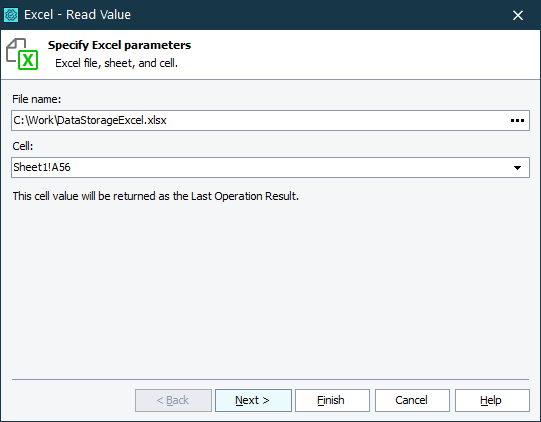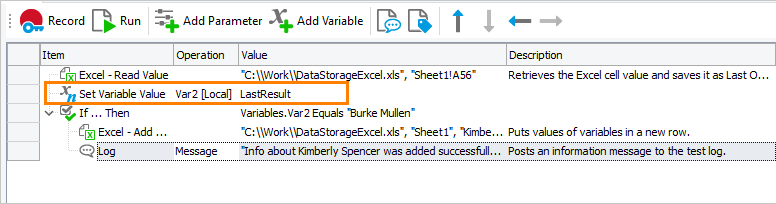When testing, you may need to read data from Excel files and then use them in your test. The recommended approach depends on your test type:

### In keyword tests

#### Operations

Note: To work with Excel files in your tests, you do not need to have Microsoft Office Excel installed on your computer.

To read data from the specified Excel cell, use the Excel - Read Value operation as described below:

1. Add the Excel - Read Value operation to your keyword test. TestComplete will display the Operation Parameters wizard.

2. On the first page of the wizard, specify Excel parameters.

• In the File Name edit box, click the ellipsis button. In the Value edit box of the subsequent dialog, specify the fully qualified file name of your Excel file.

Click OK.

• In the Cell edit box, select a cell from which data will be read. If the file is loaded correctly, the Cell edit box displays the content of the file, and you can choose one of the existing cells. Otherwise, you can enter a cell identifier manually, for instance, Sheet1!B3.Click Next.

3. On the next page of the wizard, review the parameters you have created and change them if needed.

Save the received data into a variable. For this purpose, select Last Operation Result when you specify the variable value.#### DB Table variables

 Note: Microsoft Office Excel must be installed on your computer. For more information, see Requirements for Working With Microsoft Office Excel.

To access data stored in Excel files, you can use DB Table variables. These variables store links to Excel files and provide serial access to data rows stored in these files.

You can create variables:

• On the Variables pages of the Project and ProjectSuite editors.

– or –

• When adding specific test operations to a test, for example, the Data-Driven Loop operation.

Typically, in keyword tests, you use the Data-Driven Loop operation to iterate through Excel file rows. To learn how to use the Data-Driven Loop operation in your tests, see Creating Data-Driven Loops and Data-Driven Testing With Keyword Tests - Tutorial.

### In script tests

#### Excel object

Note: To work with Excel files in your tests, you do not need to have Microsoft Office Excel installed on your computer.

To read data from Excel cells, use the `Excel` runtime object.

JavaScript, JScript

function ExcelExample()
{
// Get the sheet of the Excel file
var excelFile = Excel.Open("C:\\temp\\DataStorageExcel.xlsx");
var excelSheet = excelFile.SheetByTitle("Sheet1");

// Read data from the Excel file
var valueA = excelSheet.Cell("A", 3).Value;
var valueB = excelSheet.Cell(2, 3).Value;
var valueC = excelSheet.CellByName("C3").Value;

// Write the obtained data into a new row of the file
var rowIndex = excelSheet.RowCount + 1;
excelSheet.Cell("A", rowIndex).Value = valueA;
excelSheet.Cell(2, rowIndex).Value = valueB;
excelSheet.Cell("C", rowIndex).Value = valueC;

// Save the file to apply the changes
excelFile.Save();

// Save the file with another name
// excelFile.SaveAs("C:\\temp\\DataStorageExcel_new.xlsx");
}

Python

``````def ExcelExample():

# Get the sheet of the Excel file
excelFile = Excel.Open("C:\\temp\\DataStorageExcel.xlsx")
excelSheet = excelFile.SheetByTitle["Sheet1"]

# Read data from the Excel file
valueA = excelSheet.Cell["A", 3].Value
valueB = excelSheet.Cell[2, 3].Value
valueC = excelSheet.CellByName["C3"].Value

# Write the obtained data into a new row of the file
rowIndex = excelSheet.RowCount + 1
excelSheet.Cell["A", rowIndex].Value = valueA
excelSheet.Cell[2, rowIndex].Value = valueB
excelSheet.Cell("C", rowIndex).Value = valueC

# Save the file to apply the changes
excelFile.Save()

# Save the file with another name
# excelFile.SaveAs("C:\\temp\\DataStorageExcel_new.xlsx")``````

VBScript

Sub ExcelExample
Dim excelFile, excelSheet, valueA, valueB, valueC, rowIndex

' Get the sheet of the Excel file
Set excelFile = Excel.Open("C:\temp\DataStorageExcel.xlsx")
Set excelSheet = excelFile.SheetByTitle("Sheet1")

' Read data from the Excel file
valueA = excelSheet.Cell("A", 3).Value
valueB = excelSheet.Cell(2, 3).Value
valueС = excelSheet.CellByName("C3").Value

' Write the obtained data into a new row of the file
rowIndex = excelSheet.RowCount + 1
excelSheet.Cell("A", rowIndex).Value = valueA
excelSheet.Cell(2, rowIndex).Value = valueB
excelSheet.Cell("C", rowIndex).Value = valueC

' Save the file to apply the changes
excelFile.Save()

' Save the file with another name
' excelFile.SaveAs("C:\temp\DataStorageExcel_new.xlsx")
End Sub

DelphiScript

procedure ExcelExample;
var
excelFile, excelSheet, valueA, valueB, valueC, rowIndex;
begin
// Get the sheet of the Excel file
excelFile := Excel.Open('C:\\temp\\DataStorageExcel.xlsx');
excelSheet := excelFile.SheetByTitle('Sheet1');

// Read data from the Excel file
valueA := excelSheet.Cell('A', 3).Value;
valueB := excelSheet.Cell(2, 3).Value;
valueC := excelSheet.CellByName('C3').Value;

// Write the obtained data into a new row of the file
rowIndex := excelSheet.RowCount + 1;
excelSheet.Cell('A', rowIndex).Value := valueA;
excelSheet.Cell(2, rowIndex).Value := valueB;
excelSheet.Cell('C', rowIndex).Value := valueC;

// Save the file to apply the changes
excelFile.Save();

// Save the file with another name
// excelFile.SaveAs('C:\\temp\\DataStorageExcel_new.xlsx');
end;

C++Script, C#Script

function ExcelExample()
{
// Get the sheet of the Excel file
var excelFile = Excel["Open"]("C:\\temp\\DataStorageExcel.xlsx");
var excelSheet = excelFile["SheetByTitle"]("Sheet1");

// Read data from the Excel file
var valueA = excelSheet["Cell"]("A", 3)["Value"];
var valueB = excelSheet["Cell"](2, 3)["Value"];
var valueC = excelSheet["CellByName"]("C3")["Value"];

// Write the obtained data into a new row of the file
var rowIndex = excelSheet["RowCount + 1"];
excelSheet["Cell"]("A", rowIndex)["Value"] = valueA;
excelSheet["Cell"](2, rowIndex)["Value"] = valueB;
excelSheet["Cell"]("C", rowIndex)["Value"] = valueC;

// Save the file to apply the changes
excelFile["Save"]();

// Save the file with another name
// excelFile["SaveAs"]("C:\\temp\\DataStorageExcel.xlsx");
}

In some advanced cases, for instance, when you work with ranges of cells in Excel files, you can use the `Excel.Application` COM object.

 Note: Microsoft Office Excel must be installed on your computer.

#### DDTDriver object

 Note: Microsoft Office Excel must be installed on your computer. For more information, see Requirements for Working With Microsoft Office Excel.

The `DDTDriver` object simplifies retrieving data from Excel files and iterating through data rows in your scripts. It represents Excel data in the form of a table, where the number of its columns and rows is specified by the number of columns and rows of the Excel file. Using properties and methods of the `DDTDriver` object, you can get the number of columns and rows in the Excel file, obtain the value of the specified cell, etc.

The code below illustrates the use of `DDT.ExcelDriver`. This code creates a DDT driver for an Excel sheet, runs through records of the driver’s table and posts values stored in record fields to the test log:

JavaScript, JScript

var RecNo;

// Posts data to the log (helper routine)
function ProcessData()
{
var Fldr, i;

Fldr = Log.CreateFolder("Record: " + aqConvert.VarToStr(RecNo));
Log.PushLogFolder(Fldr);

for(i = 0; i < DDT.CurrentDriver.ColumnCount; i++)
Log.Message(DDT.CurrentDriver.ColumnName(i) + ": " + aqConvert.VarToStr(DDT.CurrentDriver.Value(i)));

Log.PopLogFolder();
RecNo = RecNo + 1;
}

// Creates the driver (main routine)
function TestDriver()
{
var Driver;

// Creates the driver
// If you connect to an Excel 2007 sheet, use the following method call:
// Driver = DDT.ExcelDriver("C:\\MyFile.xlsx", "Sheet1", true);
Driver = DDT.ExcelDriver("C:\\MyFile.xls", "Sheet1");

// Iterates through records
RecNo = 0;
while (! Driver.EOF() )
{
ProcessData(); // Processes data
Driver.Next(); // Goes to the next record
}

// Closing the driver
DDT.CloseDriver(Driver.Name);
}

Python

``````RecNo = 0

# Posts data to the log (helper routine)
def ProcessData():
global RecNo
Fldr = Log.CreateFolder("Record: " + aqConvert.VarToStr(RecNo))
Log.PushLogFolder(Fldr)

for i in range(DDT.CurrentDriver.ColumnCount):
Log.Message(DDT.CurrentDriver.ColumnName[i] + ": " + aqConvert.VarToStr(DDT.CurrentDriver.Value[i]))

Log.PopLogFolder()
RecNo = RecNo + 1

# Creates the driver (main routine)
def TestDriver():

# Creates the driver
# If you connect to an Excel 2007 sheet, use the following method call:
# Driver = DDT.ExcelDriver("C:\\MyFile.xlsx", "Sheet1", True)
Driver = DDT.ExcelDriver("C:\\MyFile.xls", "Sheet1")

global RecNo
# Iterates through records
RecNo = 0
while not Driver.EOF():
ProcessData() # Processes data
Driver.Next() # Goes to the next record

# Closing the driver
DDT.CloseDriver(Driver.Name)``````

VBScript

Dim RecNo

' Posts data to the log (helper routine)
Sub ProcessData
Dim Fldr, i

Fldr = Log.CreateFolder("Record: " + aqConvert.VarToStr(RecNo))
Log.PushLogFolder Fldr

For i = 0 To DDT.CurrentDriver.ColumnCount - 1
Log.Message DDT.CurrentDriver.ColumnName(i) + ": " + aqConvert.VarToStr(DDT.CurrentDriver.Value(i))
Next

Log.PopLogFolder
RecNo = RecNo + 1
End Sub

' Creates the driver (main routine)
Sub TestDriver
Dim Driver

' Creates the driver
' If you connect to an Excel 2007 sheet, use the following method call:
' Set Driver = DDT.ExcelDriver("C:\MyFile.xlsx", "Sheet1", True)
Set Driver = DDT.ExcelDriver("C:\MyFile.xls", "Sheet1")

' Iterates through records
RecNo = 0
While Not Driver.EOF
Call ProcessData() ' Processes data
Call Driver.Next() ' Goes to the next record
WEnd

' Closing the driver
Call DDT.CloseDriver(Driver.Name)
End Sub

DelphiScript

var RecNo;

// Posts data to the log (helper routine)
procedure ProcessData;
var
Fldr, i : OleVariant;
begin
Fldr := Log.CreateFolder('Record: ' + aqConvert.VarToStr(RecNo));
Log.PushLogFolder(Fldr);

for i := 0 to DDT.CurrentDriver.ColumnCount - 1 do
Log.Message(DDT.CurrentDriver.ColumnName[i] + ': ' + aqConvert.VarToStr(DDT.CurrentDriver.Value[i]));

Log.PopLogFolder;
RecNo := RecNo + 1;
end;

// Creates the driver (main routine)
procedure TestDriver;
var
Driver : OleVariant;
begin
// Creates the driver
// If you connect to an Excel 2007 sheet, use the following method call:
// Driver := DDT.ExcelDriver('C:\MyFile.xlsx', 'Sheet1', true);
Driver := DDT.ExcelDriver('C:\MyFile.xls', 'Sheet1');

// Iterates through records
RecNo := 0;
while not Driver.EOF do
begin
ProcessData; // Processes data
Driver.Next; // Goes to the next record
end;

// Closing the driver
DDT.CloseDriver(Driver.Name);
end;

C++Script, C#Script

var RecNo;

// Posts data to the log (helper routine)
function ProcessData()
{
var Fldr, i;

Fldr = Log["CreateFolder"]("Record: " + aqConvert.VarToStr(RecNo));
Log["PushLogFolder"](Fldr);

for(i = 0; i < DDT["CurrentDriver"]["ColumnCount"]; i++)
Log.Message(DDT["CurrentDriver"]["ColumnName"](i) + ": " + aqConvert.VarToStr(DDT["CurrentDriver"]["Value"](i)));

Log["PopLogFolder"]();
RecNo = RecNo + 1;
}

// Creates the driver (main routine)
function TestDriver()
{
var Driver;

// Creates the driver
// If you connect to an Excel 2007 sheet, use the following method call:
// Driver = DDT["ExcelDriver"]("C:\\MyFile.xlsx", "Sheet1", true);
Driver = DDT["ExcelDriver"]("C:\\MyFile.xls", "Sheet1");

// Iterates through records
RecNo = 0;
while (! Driver["EOF"]() )
{
ProcessData(); // Processes data
Driver["Next"](); // Goes to the next record
}

// Closing the driver
DDT["CloseDriver"](Driver["Name"]);
}

 Note: There are some specifics of using the `DDTDriver` scripting object to read data from Excel worksheets. For more information about this, see Using Excel Files as Data Storages.

You can also use the `DDTDriver` object in keyword tests. In this case, to call the `DDT.ExcelDriver` method and properties and methods of the returned object, use the Run Code Snippet and Call Object Method operations. However, the best way to access Excel data from keyword tests is to use DB Table variables. For more information about this, see Using DB Table Variables to Retrieve Data From Excel Files.

#### DB Table variables

 Note: Microsoft Office Excel must be installed on your computer. For more information, see Requirements for Working With Microsoft Office Excel.

To access data stored in Excel files, you can use DB Table variables. These variables store links to Excel files and provide serial access to data rows stored in these files. To create a new DB Table variable, use the Variables pages of the Project and ProjectSuite editors.